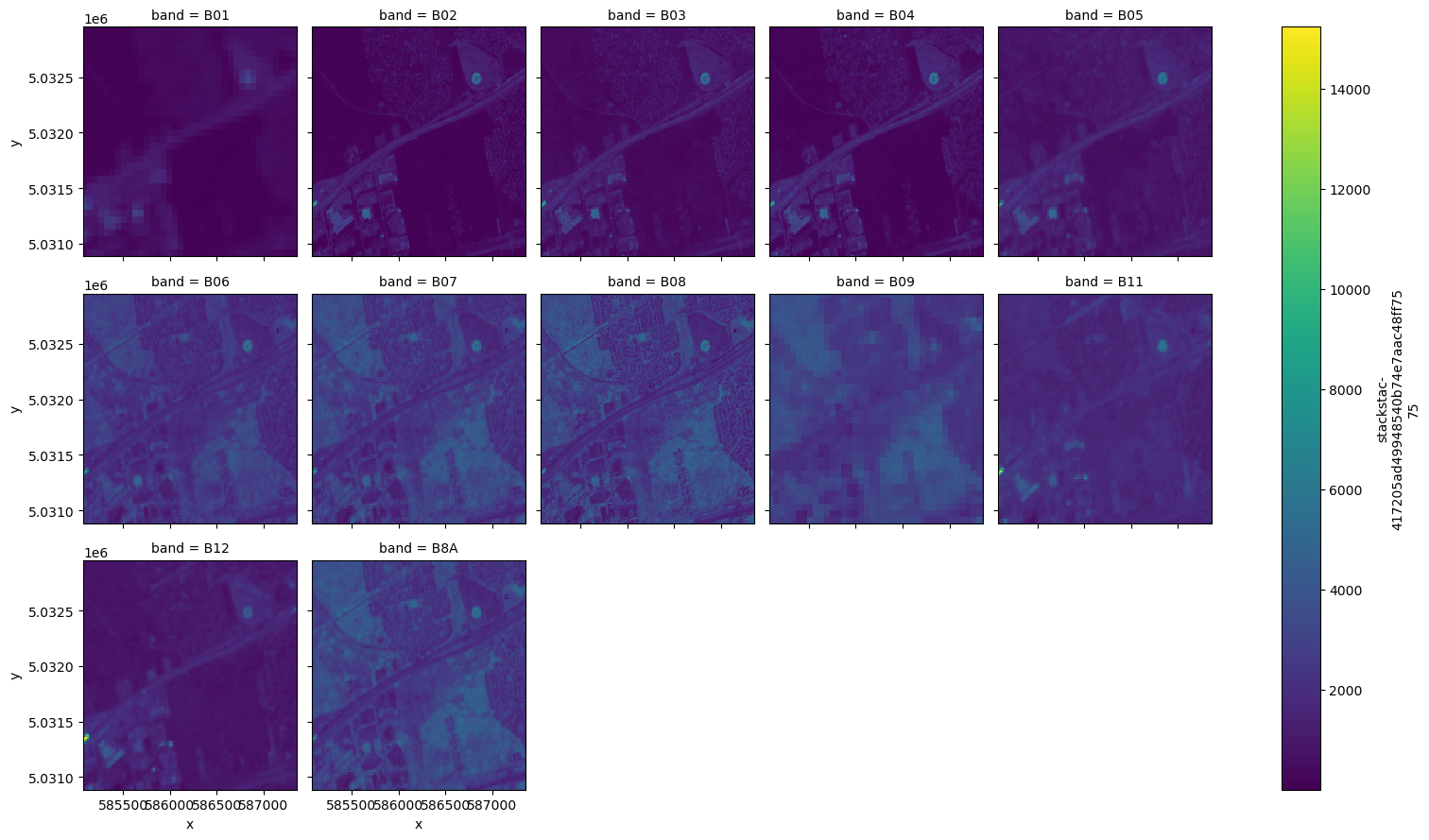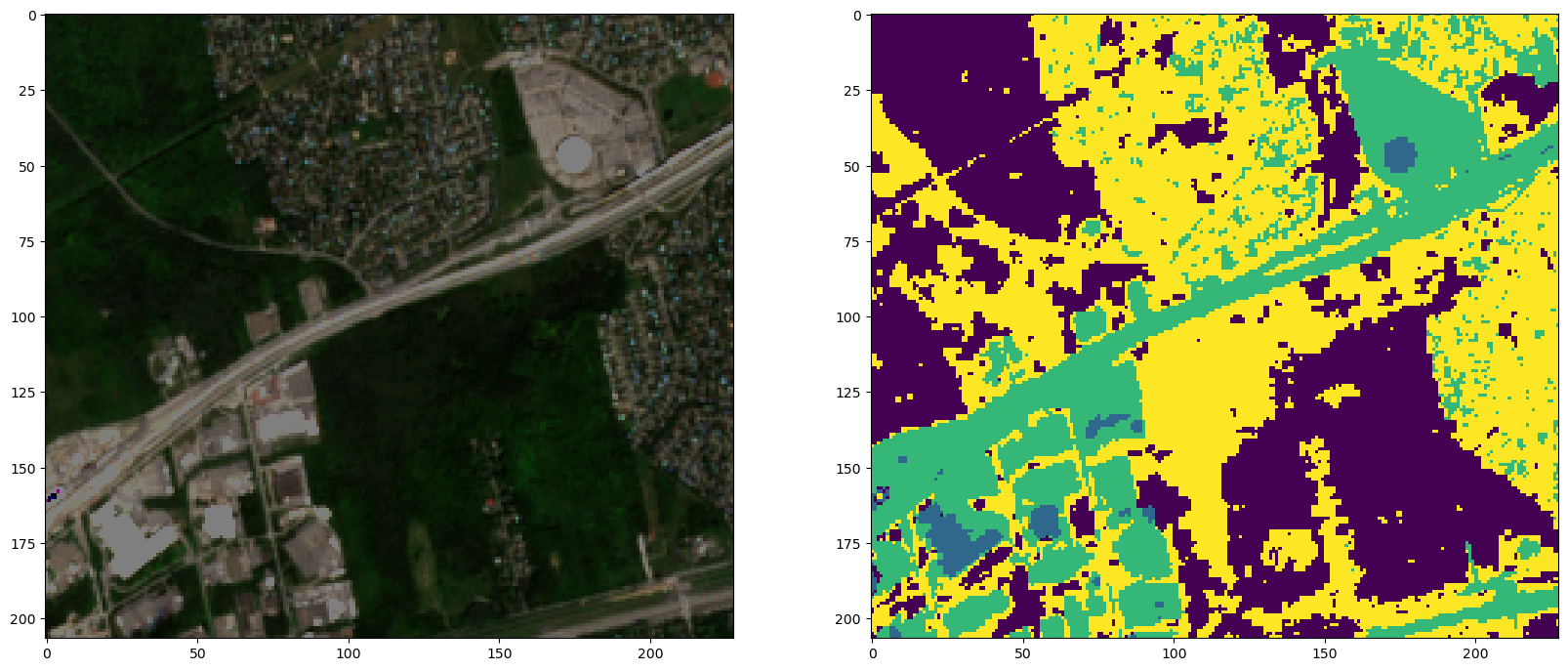# K-Means Land Classification with Dask#

K-Means is a clustering algorithm that creates a segmentation map of different “clusters” which can represent estimated/easily-separable classifications which share similar values to a centroid optimum that represents the groups mean value. The classifications should not be considered accurate and requires verification - however it is a great starting point for unsupervised classification problems to determine separable classes.

For geospatial applications, we can use K-Means to create rough land-classification segmentation maps or generate automated labeled data given supporting methods to verify the classification is correct.

[ ]:

# We will be using Sentinel-2 L2A imagery from Microsoft Planetary Computer STAC server:
!pip install planetary_computer

[ ]:

import os
import rasterio
import rioxarray
import pystac
import stackstac
import datetime
import planetary_computer
import json
import gcsfs

import numpy as np
import xarray as xr
import rioxarray as rxr
import matplotlib.pyplot as plt
import geopandas as gpd

from skimage.exposure import rescale_intensity
from shapely.geometry import Polygon
from pystac_client import Client


We will use Dask to power our computations of a K-Means algorithm with which will be fitted and used to for predictions. Start by initializing a dask cluster in a separate notebook and connecting to it. We then scaled our cluster to have 3 workers.

Remember to replace the dask cluster’s name below with the one you instantiate.

:

gateway = Gateway()
client = cluster.get_client()
cluster


## AOI#

This AOI was generated from: https://www.keene.edu/campus/maps/tool/

We will, for the purpose of this demonstration, look at the Timberlea suburb in Montreal, Quebec, Canada

:

_polygon = {
"coordinates": [
[
[
-73.8847303,
45.4294192
],
[
-73.883357,
45.4445361
],
[
-73.9108229,
45.4442049
],
[
-73.9120245,
45.4263471
],
[
-73.8847303,
45.4294192
]
]
],
"type": "Polygon"
}

:

lon_list = []
lat_list = []

for lon,lat in _polygon['coordinates']:
lon_list.append(lon)
lat_list.append(lat)
polygon_geom = Polygon(zip(lon_list, lat_list))
crs = 'EPSG:4326'
polygon = gpd.GeoDataFrame(index=, crs=crs, geometry=[polygon_geom])

:

# Set up Stac Client
api = Client.open('https://planetarycomputer.microsoft.com/api/stac/v1')
api

:

:

# CONFIG
# -------------
FOOTPRINT = polygon.to_crs('epsg:4326').geometry.envelope
TGT_BANDS =  ['B01', 'B02', 'B03', 'B04', 'B05', 'B06', 'B07', 'B08', 'B09', 'B11', 'B12', 'B8A']
YEAR = '2021'
BEGIN_MONTH = '07'
END_MONTH = '08'
DATE_RANGE = f'{YEAR}-{BEGIN_MONTH}-01/{YEAR}-{END_MONTH}-30'
MAX_CLOUD = 5
RESOLUTION = 10
# -------------

:

# In our AOI with a max cloud cover of 5%, we find one image
items = api.search(
collections = ['sentinel-2-l2a'],
intersects = FOOTPRINT,
query={"eo:cloud_cover": {"lt": MAX_CLOUD}},
datetime = DATE_RANGE,
).get_all_items()

print(f'\tFound {len(items)} items')

        Found 1 items

:

# Planetary Computer requires signing the items HREF so we can pull the Asset
signed_items = [planetary_computer.sign(item).to_dict() for item in items]

:

# Create an Xarray DataArray of the pystac_client query results
data = (
stackstac.stack(
signed_items,
assets=TGT_BANDS,
resolution=RESOLUTION, # Set all bands res to this
bounds_latlon=FOOTPRINT.bounds, # clip to AOI bounds
).where(lambda x: x > 0, other=np.nan) # Convert nodata zero to np.nan
)
data

/srv/conda/envs/notebook/lib/python3.10/site-packages/stackstac/prepare.py:363: UserWarning: The argument 'infer_datetime_format' is deprecated and will be removed in a future version. A strict version of it is now the default, see https://pandas.pydata.org/pdeps/0004-consistent-to-datetime-parsing.html. You can safely remove this argument.
times = pd.to_datetime(

:

<xarray.DataArray 'stackstac-417205ad49948540b74e7aac48ff7575' (time: 1,
band: 12,
y: 207, x: 228)>
dask.array<where, shape=(1, 12, 207, 228), dtype=float64, chunksize=(1, 1, 207, 228), chunktype=numpy.ndarray>
Coordinates: (12/44)
* time                                     (time) datetime64[ns] 2021-08-03...
id                                       (time) <U54 'S2A_MSIL2A_20210803...
* band                                     (band) <U3 'B01' 'B02' ... 'B8A'
* x                                        (x) float64 5.851e+05 ... 5.874e+05
* y                                        (y) float64 5.033e+06 ... 5.031e+06
s2:product_uri                           <U65 'S2A_MSIL2A_20210803T154911...
...                                       ...
title                                    (band) <U37 'Band 1 - Coastal ae...
gsd                                      (band) float64 60.0 10.0 ... 20.0
common_name                              (band) object 'coastal' ... 'red...
center_wavelength                        (band) float64 0.443 0.49 ... 0.865
full_width_half_max                      (band) float64 0.027 ... 0.033
epsg                                     int64 32618
Attributes:
spec:        RasterSpec(epsg=32618, bounds=(585080, 5030880, 587360, 5032...
crs:         epsg:32618
transform:   | 10.00, 0.00, 585080.00|\n| 0.00,-10.00, 5032950.00|\n| 0.0...
resolution:  10
:

# Visualize each band
data.plot.imshow(x='x', y='y', col='band', col_wrap=5)

:

<xarray.plot.facetgrid.FacetGrid at 0x7f6de6a84b20>We are going to normalize our data and shift it into a int8 [0, 255] scale.

:

def normalize(array):
norm = ((array - array.min()) / (array.max() - array.min())*255).astype(np.int8)
return norm

:

data_norm = normalize(data)
data_norm

:

<xarray.DataArray 'stackstac-417205ad49948540b74e7aac48ff7575' (time: 1,
band: 12,
y: 207, x: 228)>
dask.array<astype, shape=(1, 12, 207, 228), dtype=int8, chunksize=(1, 1, 207, 228), chunktype=numpy.ndarray>
Coordinates: (12/44)
* time                                     (time) datetime64[ns] 2021-08-03...
id                                       (time) <U54 'S2A_MSIL2A_20210803...
* band                                     (band) <U3 'B01' 'B02' ... 'B8A'
* x                                        (x) float64 5.851e+05 ... 5.874e+05
* y                                        (y) float64 5.033e+06 ... 5.031e+06
s2:product_uri                           <U65 'S2A_MSIL2A_20210803T154911...
...                                       ...
title                                    (band) <U37 'Band 1 - Coastal ae...
gsd                                      (band) float64 60.0 10.0 ... 20.0
common_name                              (band) object 'coastal' ... 'red...
center_wavelength                        (band) float64 0.443 0.49 ... 0.865
full_width_half_max                      (band) float64 0.027 ... 0.033
epsg                                     int64 32618
:

# Visualize the normalized data and see that it's visually the same as before
data_norm.plot.imshow(x='x', y='y', col='band', col_wrap=5, vmin=0, vmax=255)

:

<xarray.plot.facetgrid.FacetGrid at 0x7f6de43faec0>## Initialize K-Means Algorithm#

:

km = dask_ml.cluster.KMeans(n_clusters=4, oversampling_factor=0)
km

:

KMeans(n_clusters=4, oversampling_factor=0)
In a Jupyter environment, please rerun this cell to show the HTML representation or trust the notebook.

## Data Preprocessing#

Start by figuring out the shape of our data. Doing so will give us a better understanding of how to manipulate the data for the algorithm.

:

arr_shape = data.shape
arr_shape

:

(1, 12, 207, 228)


The K-Means algorithm requires a 2-dimensional array as an input. First we will essentially flatten the bands invidiually and then we will transpose the array so that each “column” represents a band.

:

arr = data_norm.data.reshape(arr_shape, arr_shape*arr_shape).T
arr

:

 Array Chunk 553.08 kiB 46.09 kiB (47196, 12) (47196, 1) 12 chunks in 25 graph layers int8 numpy.ndarray

Make sure the entire array is visible to the K-Means algorithm (not as chunks - you will get Errors)

:

arr_rc = arr.rechunk({1: arr.shape})
arr_rc

:

 Array Chunk 553.08 kiB 553.08 kiB (47196, 12) (47196, 12) 1 chunks in 26 graph layers int8 numpy.ndarray

## Fitting The K-Means Algorithm#

Here we will fit our input AOI imagery in to the K-Means algorithm

:

%%time
km.fit(arr_rc)

Found fewer than 4 clusters in init (found 1).

CPU times: user 2.48 s, sys: 105 ms, total: 2.59 s
Wall time: 30.2 s

:

KMeans(n_clusters=4, oversampling_factor=0)
In a Jupyter environment, please rerun this cell to show the HTML representation or trust the notebook.

## Predicting Our Classification Clusters#

Once fitted, we can then perform predictions based on the calculated centroids of the input AOI imagery. For simplicity, we are using the same input for both fitting and prediction. Some fitted algorithms can be extended to similar areas - too dissimilar then the results will not be confident.

:

%%time
pred = km.predict(arr_rc)
pred

CPU times: user 22.1 ms, sys: 459 µs, total: 22.6 ms
Wall time: 371 ms

:

 Array Chunk 184.36 kiB 184.36 kiB (47196,) (47196,) 1 chunks in 5 graph layers int32 numpy.ndarray

To visualize the data, we will need to reverse the steps we performed to the input. So, we will transpose and then reshape back into the original X and Y dimensions.

:

pred = pred.T.reshape(arr_shape, arr_shape)
pred

:

 Array Chunk 184.36 kiB 184.36 kiB (207, 228) (207, 228) 1 chunks in 7 graph layers int32 numpy.ndarray

Below is an RGB (left) and K-Means Cluster Prediction segmenation map (right) of our input AOI

:

fig, ax = plt.subplots(1, 2, figsize=(20,10))
ax.imshow(rescale_intensity(data_norm.sel(band=['B04','B03','B02']).data, in_range=(0,30)).transpose(1,2,0))
ax.imshow(pred)

:

<matplotlib.image.AxesImage at 0x7f6ddb2aa560>As you can see, the K-Means can easily pick out major concrete infrastructure such as industry areas and highways. It also appears to do a decent job at separating different vegetation densities/types. However, in the residential/urban areas the algorithm doesn’t do as well. That could be an element of the sensor’s spatial resolution and the quality of the segmentation map could be improved on by adding more indicies (eg: NDVI) to the input/prediction operations.